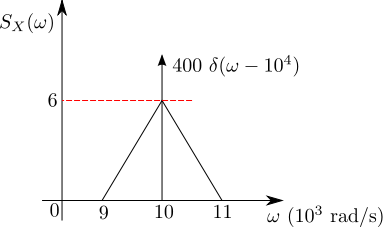# GATE-2012 ECE Q2 (communication)Question 52 on communication from GATE (Graduate Aptitude Test in Engineering) 2012 Electronics and Communication Engineering paper.

## Q2. The power spectral density of a real process for positive frequencies is shown below. The values of  and , respectively are## (D)## Solution

For a wide sense stationary function, the auto-correlation with delay  is defined as,

From the Weiner-Kinchin theorem, the auto-correlation function  and power spectral density are Fourier Transform pairs, i.e.

Expressing in terms of , where

.

When the delay , the above equations simplifies to

.

Applying this to the problem at hand,

.

Further, since the power spectral density  does not have any dc component, the mean of the signal

Based on the above, the right choice is (B)

## References

 GATE Examination Question Papers [Previous Years] from Indian Institute of Technology, Madras http://gate.iitm.ac.in/gateqps/2012/ec.pdf